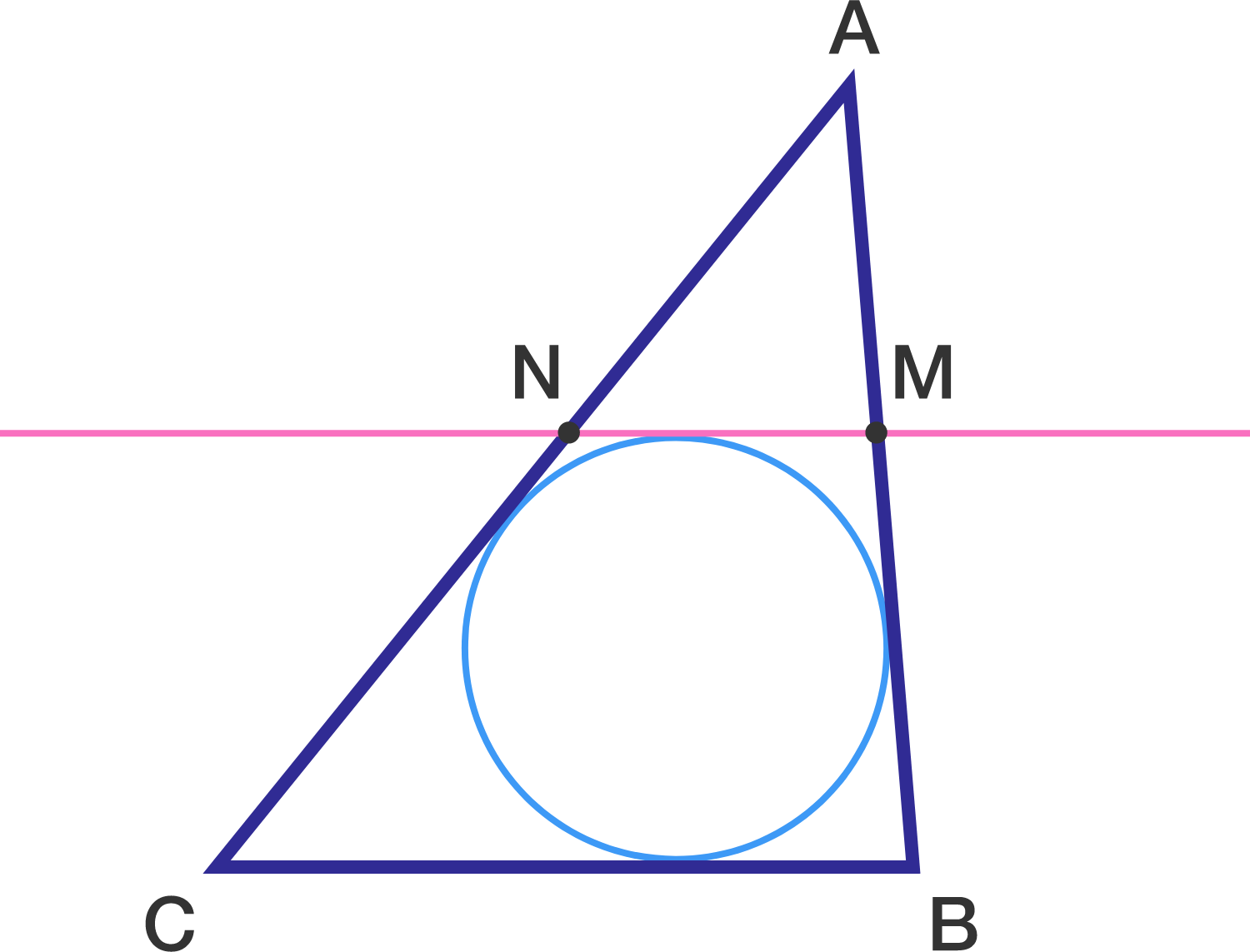# Parallel Tangent

Geometry Level 2In $\triangle ABC,$ $AB=6$ , $BC = 4$ and $AC=8.$ A segment parallel to $\overline{BC}$ and tangent to the incircle of $\triangle ABC$ intersects $\overline{AB}$ at $M$ and $\overline{AC}$ at $N$.

If $MN=\dfrac{a}{b}$, where $a$ and $b$ are co-prime positive integers, what is the value of $a+b$ ?

×